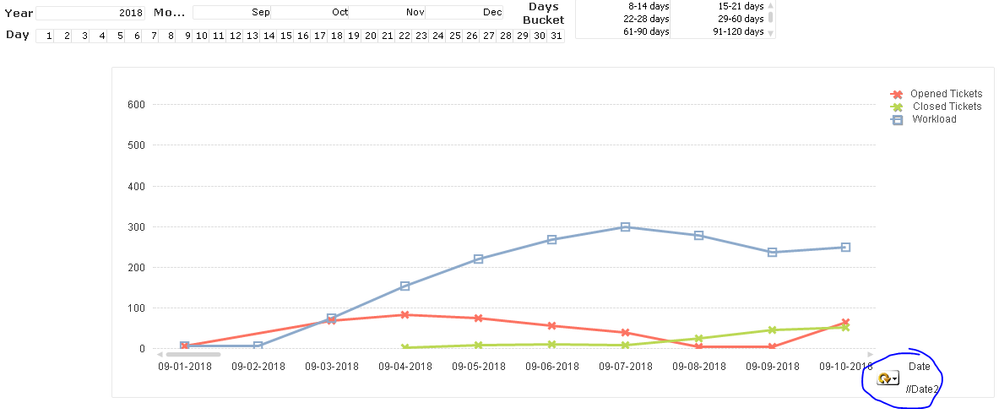# App Development

Announcements
Support Case Portal has moved to Qlik Community! Read the FAQs to start exploring Support resources.
cancel
Showing results for
Did you mean:Master III

## 30,60,90 bucket Filter help

Hello Folks,

I have created this Workload Calculation chart based on Open and Close ticket , which is working fine.When a user is doing selections from Year , Month etc workload calculation never breaks ( Carry forwards numbers from previous days).

Now I'm looking for a solution to create last 30 , 60, 90 days buckets to help users. To achieve this I created at the backend  "DaysDifferenceGroup" filed , but the bucket breaks the workload calculation, the calc is set to 0 at the beginning of the bucket period rather than the true opening value.

GenCal:
*,
dual(if(DaysDifference >=0 and DaysDifference <= 7, '0-7 days',
if(DaysDifference >=8 and DaysDifference <= 14, '8-14 days',
if(DaysDifference >=15 and DaysDifference <= 21, '15-21 days',
if(DaysDifference >=22 and DaysDifference <= 28, '22-28 days',
if(DaysDifference >=29 and DaysDifference <= 60, '29-60 days',
if(DaysDifference >=61 and DaysDifference <= 90, '61-90 days',
if(DaysDifference >=91 and DaysDifference <= 120, '91-120 days',
if(DaysDifference >121, 'Above 121 days')))))))),
if(DaysDifference >=0 and DaysDifference <= 7, 10,
if(DaysDifference >=7 and DaysDifference <= 14, 20,
if(DaysDifference >=15 and DaysDifference <= 21, 30,
if(DaysDifference >=22 and DaysDifference <= 28, 40,
if(DaysDifference >=29 and DaysDifference <= 60, 50,
if(DaysDifference >=61 and DaysDifference <= 90, 60,
if(DaysDifference >=91 and DaysDifference <= 120, 70,
if(DaysDifference >121, 80))))))))
) as DaysDifferenceGroup;

Datefield as %DateID,
\$(vToday)-floor(num(Datefield)) AS DaysDifference,
dual(date(Datefield, 'MMM YY'), year(Datefield)*100+month(Datefield)) as MonthYear,
year(Datefield) as Year,
month(Datefield) as Month,
day(Datefield) as Day,
week(Datefield) & ' / ' & year(Datefield) as Week,
weekday(Datefield) as Weekday,
'Q' & Ceil(Month(Datefield)/3) as Quarter
RESIDENT Datefield;
drop table Datefield;

Can someone help on How to create that last 30,60,90 days bucket , which should not break the Workload actual calculation on selections.

Attached is the QVW.

Regards,

AS

Labels (2)

• ### Script

0 RepliesTags
Community Browser